# Linear programming integer linear programming mixed integer linear

• Slides: 15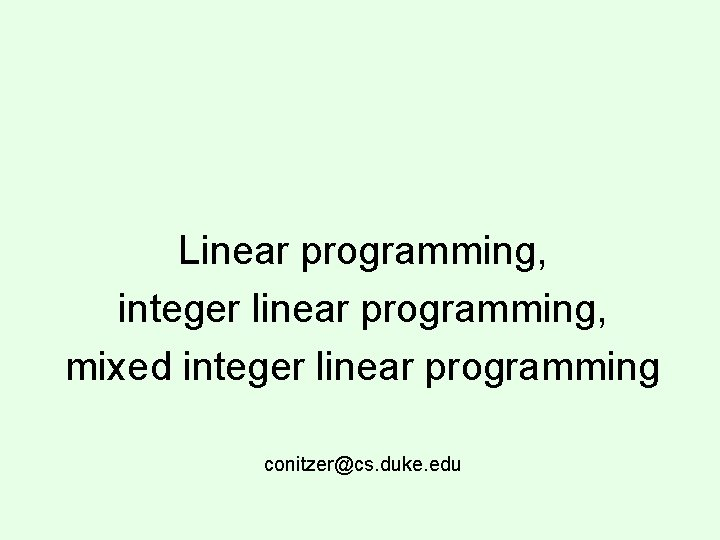Linear programming, integer linear programming, mixed integer linear programming [email protected] duke. edu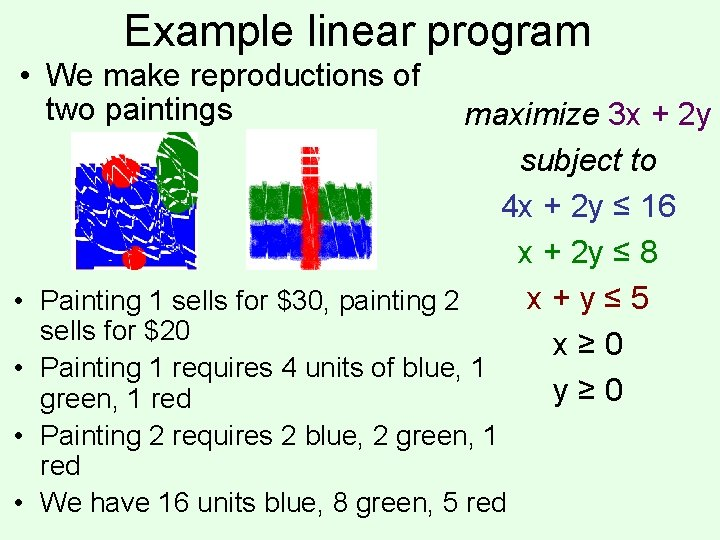Example linear program • We make reproductions of two paintings maximize 3 x + 2 y subject to 4 x + 2 y ≤ 16 x + 2 y ≤ 8 x+y≤ 5 • Painting 1 sells for \$30, painting 2 sells for \$20 x≥ 0 • Painting 1 requires 4 units of blue, 1 y≥ 0 green, 1 red • Painting 2 requires 2 blue, 2 green, 1 red • We have 16 units blue, 8 green, 5 red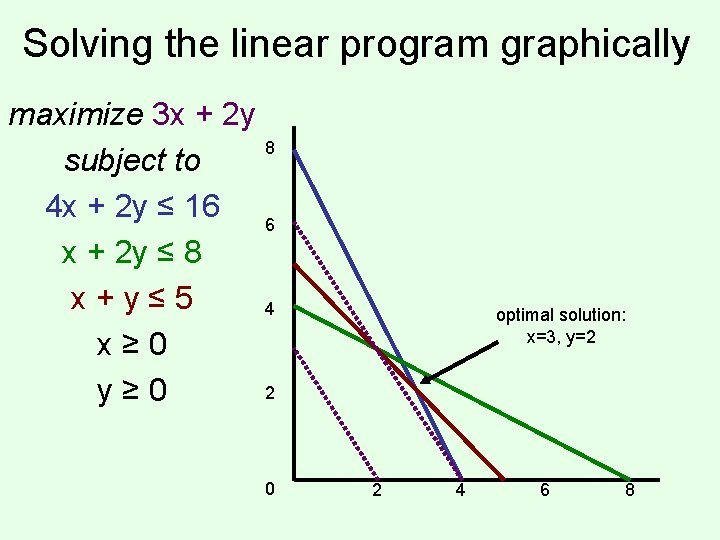Solving the linear program graphically maximize 3 x + 2 y 8 subject to 4 x + 2 y ≤ 16 6 x + 2 y ≤ 8 x+y≤ 5 4 x≥ 0 2 y≥ 0 0 optimal solution: x=3, y=2 2 4 6 8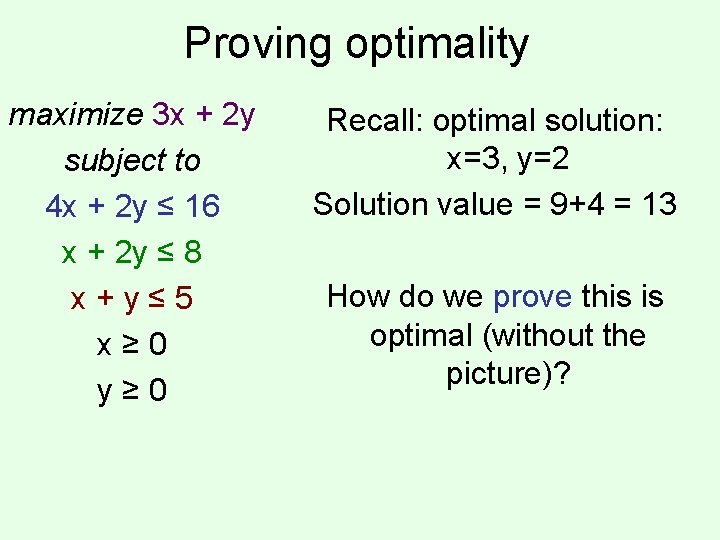Proving optimality maximize 3 x + 2 y subject to 4 x + 2 y ≤ 16 x + 2 y ≤ 8 x+y≤ 5 x≥ 0 y≥ 0 Recall: optimal solution: x=3, y=2 Solution value = 9+4 = 13 How do we prove this is optimal (without the picture)?Proving optimality… maximize 3 x + 2 y subject to 4 x + 2 y ≤ 16 x + 2 y ≤ 8 x+y≤ 5 x≥ 0 y≥ 0 We can rewrite the blue constraint as 2 x + y ≤ 8 If we add the red constraint x+y≤ 5 we get 3 x + 2 y ≤ 13 Matching upper bound! (Really, we added. 5 times the blue constraint to 1 times the red constraint)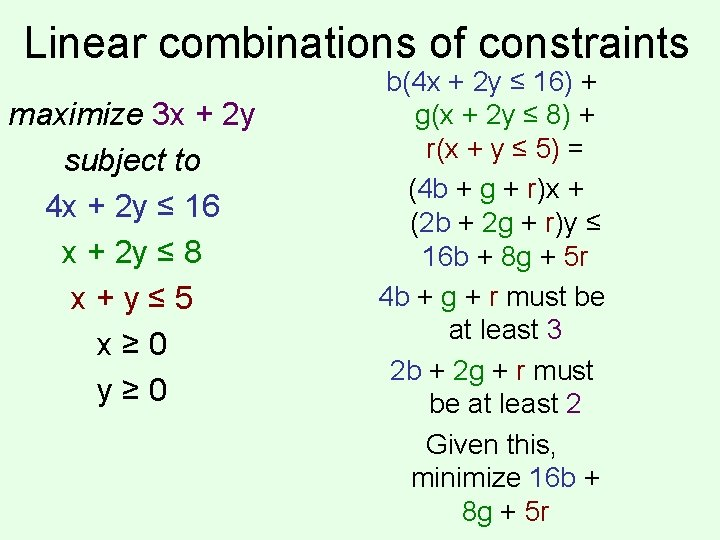Linear combinations of constraints maximize 3 x + 2 y subject to 4 x + 2 y ≤ 16 x + 2 y ≤ 8 x+y≤ 5 x≥ 0 y≥ 0 b(4 x + 2 y ≤ 16) + g(x + 2 y ≤ 8) + r(x + y ≤ 5) = (4 b + g + r)x + (2 b + 2 g + r)y ≤ 16 b + 8 g + 5 r 4 b + g + r must be at least 3 2 b + 2 g + r must be at least 2 Given this, minimize 16 b + 8 g + 5 rUsing LP for getting the best upper bound on an LP maximize 3 x + 2 y subject to 4 x + 2 y ≤ 16 x + 2 y ≤ 8 x+y≤ 5 x≥ 0 y≥ 0 minimize 16 b + 8 g + 5 r subject to 4 b + g + r ≥ 3 2 b + 2 g + r ≥ 2 b≥ 0 g≥ 0 r≥ 0 the dual of the original program • Duality theorem: any linear program has the same optimal value as its dual!Modified LP maximize 3 x + 2 y subject to 4 x + 2 y ≤ 15 x + 2 y ≤ 8 x+y≤ 5 x≥ 0 y≥ 0 Optimal solution: x = 2. 5, y = 2. 5 Solution value = 7. 5 + 5 = 12. 5 Half paintings?Integer (linear) program maximize 3 x + 2 y 8 subject to 4 x + 2 y ≤ 15 6 x + 2 y ≤ 8 x+y≤ 5 4 x ≥ 0, integer y ≥ 0, integer 2 0 optimal IP solution: x=2, y=3 (objective 12) optimal LP solution: x=2. 5, y=2. 5 (objective 12. 5) 2 4 6 8Mixed integer (linear) program maximize 3 x + 2 y 8 subject to 4 x + 2 y ≤ 15 6 x + 2 y ≤ 8 x+y≤ 5 4 x≥ 0 y ≥ 0, integer 2 0 optimal IP solution: x=2, y=3 (objective 12) optimal LP solution: x=2. 5, y=2. 5 (objective 12. 5) optimal MIP solution: x=2. 75, y=2 (objective 12. 25) 2 4 6 8Solving linear/integer programs • Linear programs can be solved efficiently – Simplex, ellipsoid, interior point methods… • (Mixed) integer programs are NP-hard to solve – Quite easy to model many standard NP-complete problems as integer programs (try it!) – Search type algorithms such as branch and bound • Standard packages for solving these – GNU Linear Programming Kit, CPLEX, Gurobi, … • LP relaxation of (M)IP: remove integrality constraints – Gives upper bound on MIP (~admissible heuristic)Exercise in modeling: knapsack-type problem • • • We arrive in a room full of precious objects Can carry only 30 kg out of the room Can carry only 20 liters out of the room Want to maximize our total value Unit of object A: 16 kg, 3 liters, sells for \$11 – There are 3 units available • Unit of object B: 4 kg, 4 liters, sells for \$4 – There are 4 units available • Unit of object C: 6 kg, 3 liters, sells for \$9 – Only 1 unit available • What should we take?The general version of this knapsack IP maximize Σj pj xj subject to Σj wj xj ≤ W Σj vj xj ≤ V (for all j) xj ≤ aj (for all j) xj ≥ 0, xj integerExercise in modeling: cell phones (set cover) • We want to have a working phone in every continent (besides Antarctica) • … but we want to have as few phones as possible • Phone A works in NA, SA, Af • Phone B works in E, Af, As • Phone C works in NA, Au, E • Phone D works in SA, As, E • Phone E works in Af, As, Au • Phone F works in NA, E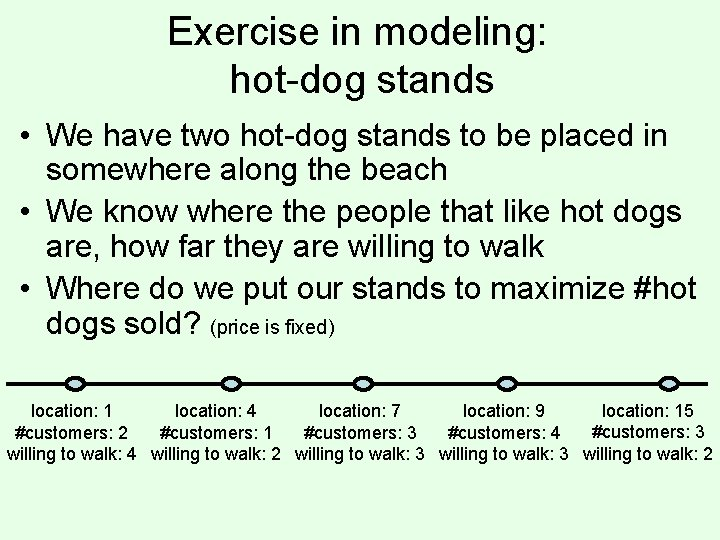Exercise in modeling: hot-dog stands • We have two hot-dog stands to be placed in somewhere along the beach • We know where the people that like hot dogs are, how far they are willing to walk • Where do we put our stands to maximize #hot dogs sold? (price is fixed) location: 15 location: 1 location: 4 location: 7 location: 9 #customers: 3 #customers: 2 #customers: 1 #customers: 3 #customers: 4 willing to walk: 2 willing to walk: 3 willing to walk: 2